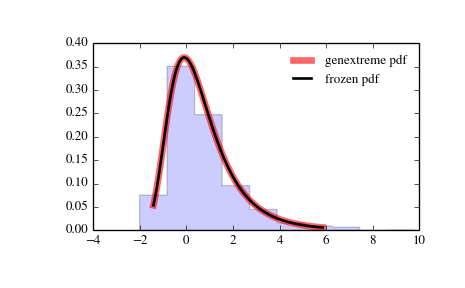# scipy.stats.genextreme¶

scipy.stats.genextreme = <scipy.stats._continuous_distns.genextreme_gen object at 0x7f6169d2e2d0>[source]

A generalized extreme value continuous random variable.

Continuous random variables are defined from a standard form and may require some shape parameters to complete its specification. Any optional keyword parameters can be passed to the methods of the RV object as given below:

Parameters: x : array_like quantiles q : array_like lower or upper tail probability c : array_like shape parameters loc : array_like, optional location parameter (default=0) scale : array_like, optional scale parameter (default=1) size : int or tuple of ints, optional shape of random variates (default computed from input arguments ) moments : str, optional composed of letters [‘mvsk’] specifying which moments to compute where ‘m’ = mean, ‘v’ = variance, ‘s’ = (Fisher’s) skew and ‘k’ = (Fisher’s) kurtosis. Default is ‘mv’. Alternatively, the object may be called (as a function) to fix the shape, location, and scale parameters returning a “frozen” continuous RV object: rv = genextreme(c, loc=0, scale=1) Frozen RV object with the same methods but holding the given shape, location, and scale fixed.

gumbel_r

Notes

For c=0, genextreme is equal to gumbel_r. The probability density function for genextreme is:

genextreme.pdf(x, c) =
exp(-exp(-x))*exp(-x),                    for c==0
exp(-(1-c*x)**(1/c))*(1-c*x)**(1/c-1),    for x <= 1/c, c > 0


Note that several sources and software packages use the opposite convention for the sign of the shape parameter c.

Examples

>>> from scipy.stats import genextreme
>>> import matplotlib.pyplot as plt
>>> fig, ax = plt.subplots(1, 1)


Calculate a few first moments:

>>> c = -0.1
>>> mean, var, skew, kurt = genextreme.stats(c, moments='mvsk')


Display the probability density function (pdf):

>>> x = np.linspace(genextreme.ppf(0.01, c),
...               genextreme.ppf(0.99, c), 100)
>>> ax.plot(x, genextreme.pdf(x, c),
...          'r-', lw=5, alpha=0.6, label='genextreme pdf')


Alternatively, freeze the distribution and display the frozen pdf:

>>> rv = genextreme(c)
>>> ax.plot(x, rv.pdf(x), 'k-', lw=2, label='frozen pdf')


Check accuracy of cdf and ppf:

>>> vals = genextreme.ppf([0.001, 0.5, 0.999], c)
>>> np.allclose([0.001, 0.5, 0.999], genextreme.cdf(vals, c))
True


Generate random numbers:

>>> r = genextreme.rvs(c, size=1000)


And compare the histogram:

>>> ax.hist(r, normed=True, histtype='stepfilled', alpha=0.2)
>>> ax.legend(loc='best', frameon=False)
>>> plt.show()Methods

 rvs(c, loc=0, scale=1, size=1) Random variates. pdf(x, c, loc=0, scale=1) Probability density function. logpdf(x, c, loc=0, scale=1) Log of the probability density function. cdf(x, c, loc=0, scale=1) Cumulative density function. logcdf(x, c, loc=0, scale=1) Log of the cumulative density function. sf(x, c, loc=0, scale=1) Survival function (1-cdf — sometimes more accurate). logsf(x, c, loc=0, scale=1) Log of the survival function. ppf(q, c, loc=0, scale=1) Percent point function (inverse of cdf — percentiles). isf(q, c, loc=0, scale=1) Inverse survival function (inverse of sf). moment(n, c, loc=0, scale=1) Non-central moment of order n stats(c, loc=0, scale=1, moments='mv') Mean(‘m’), variance(‘v’), skew(‘s’), and/or kurtosis(‘k’). entropy(c, loc=0, scale=1) (Differential) entropy of the RV. fit(data, c, loc=0, scale=1) Parameter estimates for generic data. expect(func, c, loc=0, scale=1, lb=None, ub=None, conditional=False, **kwds) Expected value of a function (of one argument) with respect to the distribution. median(c, loc=0, scale=1) Median of the distribution. mean(c, loc=0, scale=1) Mean of the distribution. var(c, loc=0, scale=1) Variance of the distribution. std(c, loc=0, scale=1) Standard deviation of the distribution. interval(alpha, c, loc=0, scale=1) Endpoints of the range that contains alpha percent of the distribution

#### Previous topic

scipy.stats.genexpon

#### Next topic

scipy.stats.gausshyper